LASTNONBLANK Explained - Excelerator BI

# LASTNONBLANK Explained

Level: Intermediate

Last week at my Sydney training course, one of the students asked me a question about LASTNONBLANK.  This reminded me what a trickily deceptive function LASTNONBLANK is.  It sounds like an easy DAX formula to understand, right?  It just finds the last non blank value in a column – easy right?  Well it is a little bit trickier than that, and I am going to explain it all in this post today.

LASTNONBLANK has a sibling FIRSTNONBLANK that operates in exactly the same way but in reverse.  Given the behaviour is the same, I won’t cover FIRSTNONBLANK at all, as you can work it out after reading about LASTNONBLANK.

## Syntax

The syntax of LASTNONBLANK is as follows.

LASTNONBLANK(Table[Column],<expression>)

It takes 2 parameters, 1) a column and 2) an expression.  In fact you can also pass a single column table as the first parameter in as well.  This could be useful for example if you wanted to create your own table on the fly using a function that returns a table, and then use that as the first parameter.

### LASTNONBLANK is an Iterator

It is not immediately obvious, but LASTNONBLANK is actually an iterator,  It iterates over the column specified in the first parameter and finds (unsurprisingly) the last value in that column that is not blank.  (Technically the engine carries out this task in reverse natural sort order for efficiency).

### But what is that pesky second parameter?

The first thing people normally find confusing about LASTNONBLANK is that pesky second parameter.  If all you want to do is find the last non blank value in a column, why do you need this second parameter?  Well in short, you don’t “need” it in that use case (except that it is mandatory).  But having this parameter makes the formula much more powerful, so it is good to have it as an option. It is designed to allow you to write a DAX expression such as a measure to test for some condition or value.  Ideally (in my view) Microsoft should have made this an optional parameter, but that is not what we got.  But there is an easy work around that allows you to make this parameter optional  – just use the value 1 as the second parameter, like this.

`myMeasure = LASTNONBLANK(Table[Column], 1)`

LASTNONBLANK iterates through the Table[Column] and then checks to see if the second parameter has a value.  The number 1 always has a value of course, so placing 1 as the second parameter has the same effect as just ignoring this parameter.  Instead the formula will just return the last non blank value it finds in the column.

### LASTNONBLANK actually returns a TABLE

Another thing that is not immediately obvious is that LASTNONBLANK actually returns a TABLE.  It is a special single column, single row table that by definition can only have 1 possible value (because it has only 1 row and 1 column).  One feature of this single row, single column table is that you can use it as both a scalar value or a Table in DAX.  More on that later too.

### Test Data

My test data for this blog post is monthly bank account balances for 2 fake bank accounts.  Measures that calculate account balances in DAX are often described as “semi-additive measures”, because you can’t just add up the values from each month to get the current balance – you need to find the latest balance to work out how much you have.  This type of data is a prime candidate to use the LASTNONBLANK formula, because LASTNONBLANK does exactly what we need to work with semi-additive measures.

I have set up the following data with some interesting features to demonstrate the behaviour of LASTNONBLANK.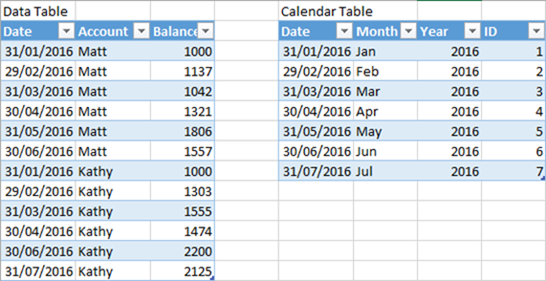It is easiest to see what I have done with this test data in the pivot table below.  I have written the following measure to demonstrate the point.

`Sum of Balance = SUM(Data[Balance])`

Note the above measure is a test measure only to help with this blog – it doesn’t really make any sense as is, but it is useful for describing what is happening.  When the above measure is placed in a pivot table, it looks like this (shown below).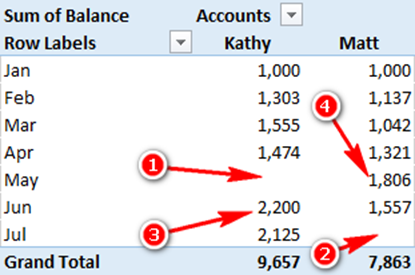1. Kathy’s account doesn’t have a result for May
2. Matt’s account doesn’t have a result for July
3. The maximum value for Kathy’s account is in June (the previous month’s data load)
4. The maximum value for Matt’s account is back in May.

I have set the data up this way to demonstrate the behaviour of LASTNONBLANK.  I often talk about how important it is to set up test data that will flush out issues when you write your formulas.  This is a good example of that, and it will make more sense as you read on.

### Last value in a Column

Now the objective is to use LASTNONBLANK to find the last value in a column.
Consider the following formula.

`Last Non Blank Date = LASTNONBLANK(Data[Date], 1)`

This formula finds the last date in the data table (note it is not the last date in the Calendar table, but the data table).

The following pivot table has the Month name from the calendar table and the account names on pivot table columns.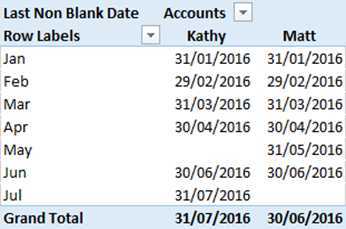Note that the formula correctly indicates that May data is missing for Kathy, and July data is missing for Matt.  Also note that it correctly gives the last date in the Grand Total of the pivot table.  (You would also get the same result as above if you used LASTDATE instead of LASTNONBLANK).  I have only used LASTNONBLANK here to demonstrate the behaviour.

### LASTNONBLANK operates over a sorted column

The [Last Non Blank Date] measure above hides some complexities about LASTNONBLANK.  You might expect that LASTNONBLANK finds the last value in the column, but that is not how it works.  It actually finds the last value in a sorted column using the natural sort behaviour of the column data type.  In the case of a date column (like shown above), then everything is sweet – the natural sort order is also the order we normally load the data (chronological order).  But in the case of the balance column, the natural sort order of a numeric column is numerical sort order, not the order the data is loaded (as you can see in the following pivot)

I have written another test measure as follows

`LastNonBlank Balance = LASTNONBLANK(Data[Balance], 1)`

When placed in the pivot table, you get the following behaviour.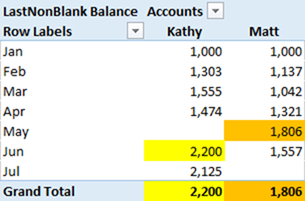In the above example, you may expect the Grand Total row to return the values 2,125 for Kathy and 1,557 for Matt as these are the last values you loaded, but that is not how it works.  When looking at the individual rows in the pivot table, it all works fine – it correctly finds the last balance for each month.  This measure works correctly on the rows in the pivot table because the rows provide “initial filter context” prior to the measure being evaluated.  So there is only ever 1 row in the data table at the time the measure is evaluated, and that is why it works in this case.  But in the Grand Total row in the pivot table, there is no initial filter context applied to the date, hence all values in the column are iterated over by LASTNONBLANK.  The iteration operates over a sorted version of the balance column (the natural sort order of the column, which is numeric in this case).  Once the balance column is sorted, then 2,200 will be the last value in the column for Kathy, and 1,806 will be the last value in the column for Matt, and that is the result that is returned.### LASTNONBLANK as a TABLE

In this next test measure, I have used LASTNONBLANK as a filter input into a CALCULATE function

```Latest Balance with 1 =
CALCULATE(
SUM(Data[Balance]),
LASTNONBLANK('Calendar'[Date], 1)
)```

You should remember that CALCULATE can use a simple filter such as Table[Column] = “some value” or it can take an advanced filter where you must pass a TABLE as the filter.  The measure above therefore suggests that LASTNONBLANK must be a table, not a scalar value.  This theory can be tested by firing up DAX Studio and executing the LASTNONBLANK portion of the formula as a query.  DAX Studio ONLY ever returns tables, it can’t return scalar values, so if the query works, it confirms that LASTNONBLANK returns a table. Note: you can read more about using DAX Studio as a tool to help you learn DAX at this blog post here.You can see above that LASTNONBLANK returns a single column, single row table that contains a single value.  It is a special table because it can also be used as a scalar value, in the same way that you can use VALUES() as a scalar value if there is only a single row returned.  In the case of the measure [Latest Balance with 1] above, I am using LASTNONBLANK as a table parameter filter inside CALCULATE.

### But how does this table act as a filter?

One feature of temporary tables in DAX is they retain a link to the data model, and filter propagation will work between the temporary table and the rest of the data model.  You should visualise this in your mind like the image below.  Imagine a new table that has spawned into the data model and has a relationship to the table where it came from (in this case it is the data table).  The filter propagation flows from the 1 side of the relationship (temporary table imagined as shown in 1 below) to the many side of the relationship (table 2 below).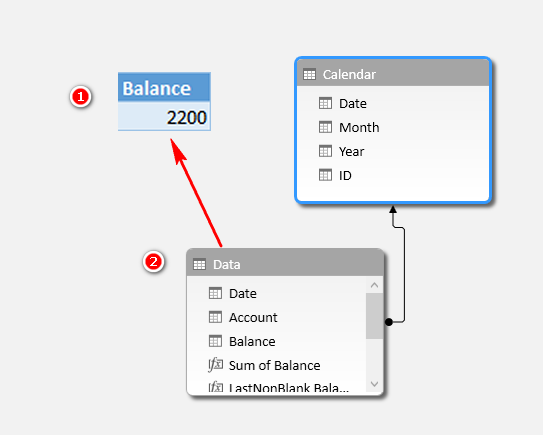So you can see above that the LASTNONBLANK function produces a table that then filters the data model based on the single value it returns in the table.

When I put this measure in a pivot table, I get this result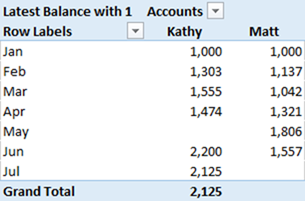Note that the measure is correctly returning the last value for the account “Kathy” but it is returning a blank for account “Matt”.  Technically this is correct because the calendar has dates in July and there is no entry for Matt for July, so the last value is blank.

### Enter the second parameter

Now it is time to change the second parameter with the following measure.

```Latest Balance with formula =
CALCULATE(
SUM(Data[Balance]),
LASTNONBLANK('Calendar'[Date], [Sum of Balance])
)```

When this last measure is added to a pivot table, it works as desired, correctly returning the last non blank value from the data table based on the chronological order of the data.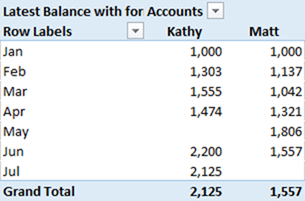This new LASTNONBLANK function measure returns the last non blank value it finds in the column provided it also returns a non-blank result for the measure [Sum of Balance].

It is worth pointing out here that the ONLY REASON this formula works is because the measure [Sum of Balance] has an implicit CALCULATE wrapped around it – you can’t see it, but it is there.  LASTNONBLANK is an iterator, and like all iterators, it has a row context but doesn’t have a filter context.  Therefore the following formula will not work

```Latest Balance with formula =
CALCULATE(
SUM(Data[Balance]),
LASTNONBLANK('Calendar'[Date], SUM(Data[Balance]))
)```

But this next measure does work (this next formula is the equivalent of the one that uses [Sum of Balance] because [Sum of Balance] has an implicit CALCULATE.

```Latest Balance with formula =
CALCULATE(
SUM(Data[Balance]),
LASTNONBLANK('Calendar'[Date],
CALCULATE(
SUM(Data[Balance])
)
)
)```

I cover evaluation context and context transition in detail in my book, Supercharge Power BI. If you want to have guided learning of the complex DAX concepts, you can enroll to my Power BI online training.### 28 thoughts on “LASTNONBLANK Explained”

1.Hello,

How should it be the formula if in addition to the current return values, I need to:

1. return values in those periods that there is no entry, i.e. to carry on the value from the previous period.
Example: May 2016, Kathy: 1,474 (rather than blank)

2. (Once point 1 is achieved) removing the “account” contextual filter so I can see the sum of all account balances at a moment in time.
e.g. balance for May 2016: 3,280 (1,474 from account “Kathy” and 1,806 from account “Matt”)

I’ve been trying to obtain this for many many hours and couldn’t manage to do it. I’m not an expert of DAX at all but couldn’t find anything clear about this despite I believe must be a usual application.

Thank you very much if any can help me with this.

Kind regards
Miguel

1.Thank you, Matt.
The article “fill table with last survey result” has helped me a lot with the first half of my case.
Then I needed to iterate that formula based on distinct values of another column.
Chat GPT did it for me!

2.Thanks, Matt. Really useful stuff.

In Power BI using LastNonBlankValue it’s clear to me how to get the one before last value, something like…
Previous Actual Value =
VAR LatestVisibleYear = MAX(FT_ACTUALS[Year ID])
CALCULATE(
LASTNONBLANKVALUE(DM_YEAR[Year], [B Actual]), DM_YEAR[Year.ID] < LatestVisibleYear )
RETURN
Result

How would you go about achieving the same in Power Pivot?
When I apply the second filter parameter to
CALCULATE ( [Measure],
LASTNONBLANK (DM_YEAR[YEAR], [Measure]),
DM_YEAR[Year.ID] < LatestVisibleYear )
No data is returned???

1.LASTNONBLANKVALUE, as the name suggests, returns the value, whereas LASTNONBLANK returns the data from the column (year, in your case)

To get the value, I normlly write

CALCULATE ( [Measure],
DM_YEAR[Year.ID] = LASTNONBLANK (DM_YEAR[YEAR], [Measure]))

If you can get that to work, you could modify it for the previous period

3.Thanks, Matt. Your blog helps a lot esp. the part about lastnonblank as an iterator.

Just want to add one point that there is not always a relationship between temporary table and your data model, the relationship only gets propagated when the 2nd parameter in lastnonblank() is wrapped with calculate or is a measure itself.

1.Yes you are correct. That is how all iterator functions operate.

4.Thanks Matt , after an hour or so of googling , your solution actually works. Will come to you first next time

5.Hi, Matt, excellent tutorial.

I have a table where I register input data very similar to the one in this post.

My target is obtain a graphic with the evolution of risk value with date.
The problem is that in the data table, I only register the date, risk ID and value when there is a change. So when I get the dinamic table with risk ID as rows, date and columns, I only get a value in those cells where there is register.

My problem is that using multiple combinations of LASTNONBLANK, SUM, FILTER, CALCULATE, I am not able to get that those empty cells show the value of last register for a risk ID.

If I do not get to fill the table, then empty cells will be 0 if I generate the graphic, when it should be a constant value up to the next register.

Could you help me to find a way to do it?
Thanks

6.Przemyslaw Remin

Hi Matt,
I try to tackle related problem. How to look up the first non blank value in unrelated table? Is it possible to rewrite the following code using FIRSTNONBLANK?

MyLookup =
var LookupKey = ThisTable[Product]
return
CALCULATE (
MAX ( SearchTable[Category] ),
SearchTable[Product] = LookupKey
)

I describe problem precisely on Stackoverflow forum: https://stackoverflow.com/q/59310106/1903793

1.I think I solved this issue:
Measure0 is measure
Measure1 is Calculated Column measure
Apple has no Category because it has 3 categories and can’t pick one, so it returns blank

Measure0 = FIRSTNONBLANK(SearchTable[Category], CALCULATE(MAX(SearchTable[Product]), TREATAS(VALUES(ThisTable[product]), SearchTable[Product])))

Measure1 = CALCULATE(FIRSTNONBLANK(SearchTable[Category],
CALCULATE( MAX(SearchTable[Product]), TREATAS(VALUES(ThisTable[product]), SearchTable[Product]))
))

7.Stephanie Giovannitti

Great article! Thank you.

8.Hi Matt

I have worked this out by adding a calculated column called CalendarDate to my Data table, that consists of RELATED(Calendar[Date]). The point being that I need to emulate the scenario where my dates only exist in the Calendar table, and I have date IDs to link to Calendar.

Last Non Blank Current Domestic Balance Date:=LASTNONBLANK ( Calendar[Date],
CALCULATE(
COUNTROWS(Data),
Data[AccountType] = “Domestic”,
Data[CalendarDate] <= TODAY())
)

Is there any better way to do this?

Thanks

1.Hi Chris. Hope you find the book useful. It is always easier to explain a problem than it is to read and understand :-). I don’t fully understand however my intuition is that you could write 2 measures – one that works in each of the scenarios and then use an if statement to detect if you are in the current month or a previous month to return the correct result. Could that work? If I have misunderstood, I suggest you create a sample workbook containing the formulas and demonstrating the problem, and then post it at http://powerpivotforum.com.au

9.Hi Matt, I liked this post so much that I bought the book! I came here while looking for a way to pass a filtered date table to LASTNONBLANK.
The setup:
I need to measure open balances in my data, where previous months are represented by just one end-of-month entry, but the current month’s open balance is represented by an entry for every day of the month, even those that are after today. I am using LASTNONBLANK to get the last day of a month, so naturally this works well for every past month, but for the current month it fetches the end-of-month date.
This is not a problem when I am only summarising my open balance, as any future date simply holds the current balance as at today, but it *is* a problem if I perform any date-based calculation (such as aging) on the data: if the LASTNONBLANK day is in the future (e.g. 31st March when today is the 7th March), and I am counting days between some past event and the current open-at-end date, this aging is off – I need to measure until the current day.
So, given this scenario, I want to restrict LASTNONBLANK to work on a filtered data set where dates are no greater than today. Furthermore, I have other filter considerations as well, such as record type. To translate this to your example imagine an additional column in the Data table named AccountType, where Matt is “Domestic” and Kathy is “Foreign”, and that the dates in the data have been brought forward to this year, 2017, meaning some of it is past (until the end of February) and the rest is future dated. And finally, I need to use the related Calendar[Date] field, rather than the date embedded in my data table, as in my case dates are joined by an integer identifier in YYYMMDD format, rather than a date (I know not best practice here but inherited from a long-standing design).
My first step is to return the correct last non-blank date no greater than today, once I have this I will use it to calculate my balance, and returning data that is not future dated.
I can get the very last non-blank Domestic sale date like this, by using the Calendar date as my expression into LASTNONBLANK:

Last Non Blank Current Domestic Balance Date:=LASTNONBLANK ( Calendar[Date],
CALCULATE(
COUNTROWS(Data),
Data[AccountType] = “Domestic”,
Data[Date] = TODAY(), therefore I cannot include that filter on Data[Date] in the CALCULATE, I think instead that I need to filter the data supplied to LASTNONBLANK. Can you show me the correct solution?

10.Great post

11.Thanks Matt – very clear !!
Just a precision : LastNonBlank performs the iteration in the reverse order.

For example, let’s imagine that we have October 2016 in the filter context. So days are sorted from 01 October 2016 to 31 October 2016. LastNonblank starts iterating from 31 October 2016 !
So if you have a balance for that day, Lastnonblank only iterated once and returns the value (as a table format as you mention) corresponding to that day (31 October 2016).

You mention that “LastNonblank returns the last non blank value if finds in the column”. Actually I would say it “returns the first last non blank value it finds in the column” since the iteration is performed in the reverse order !

1.You are right Tristan. You are talking about an engine optimisation and it makes sense to start from the bottom up. However I never actually mention which order the iteration operates in my post, and I think to do so would just add complexity that doesn’t improve the reader’s understanding.

I have added a small caveat early in the post for those that are interested.

1.Yes true, it might be a little bit confusing !

12.Hi Matt,
thanks for your response. yes, that’s exactly what i’m saying. the item is out of stock.
when I use the above calculate formula I still get the data returned for Jan. the item has been out of stock after Jan.
the following formula’s both return Jan data:
LastBalance = CALCULATE(SUM(MntEndInv[QOH]),LASTNONBLANK(dimDate[Date],CALCULATE(SUM(MntEndInv[QOH]))))
LastBalance2 = CALCULATE(SUM(MntEndInv[QOH]),LASTDATE(dimDate[Date])) (also tried using the date from the fact table, same result.)

13.I’ve used both FirstNonBlank & LastNonBlank as semi-additive measures to for a powerpivot model and most everything works as expected.
the only area that I run into issues is with items that are currently not in inventory. for example item A has inventory in months 1,2,3. then in month 4,5,6 there is no inventory,(and no inventory record in the model) so when I use “LASTNONBLANK”, the data I get is for month 3 as that is the “LASTNONBLANK” month with data. is there a way to return a blank or zero if an item hand inventory in the X previous months? but no data in the last month with out having to manually add a zero quantity for months 4,5,6 in the source data.

1.Are you saying that when a count is done, all products are counted, and if there is no count record, then it is out of stock? If so, use lastdate to find the date of the last count then use that as an input to a calculate. Something like
=calculate(sum(table[value]),lastdate(table[date]))

14.I think one more thing to consider explicitly adding is that since LASTNONBLANK (and sibling FIRSTNONBLANK ) is a table function, it does perform context transition of external row context and so need to be aware of that in certain situations. For example, My_Measure = CALCULATE( [Sum Measure], FILTER ( ALL (Calendar ), Calendar[Date] = LASTNONBLANK ( Calendar[Date],1) ) ) doesn’t return the [Sum Measure] for the last date in the Calendar, it will return [Sum Measure] for all Dates. This is because of the context transition performed by LASTNONBLANK inside the FILTER’s Calendar[Date] iteration. LASTNONBLANK ( Calendar[Date],1) will always return the currently iterated date and hence comparison always true and hence all Calendar rows returned. I’m sure you know this but food for thought to include in the blog as a potential “gotcha” for other folks when writing Dax.

15.As usual, Matt is at his best. Thanks for sharing it.

16.Hans Mostafavi

Great post. Thanks for sharing.

17.Thanks for sharing this great post Matt! Definitely a sneakier function than I thought.

Scroll to Top